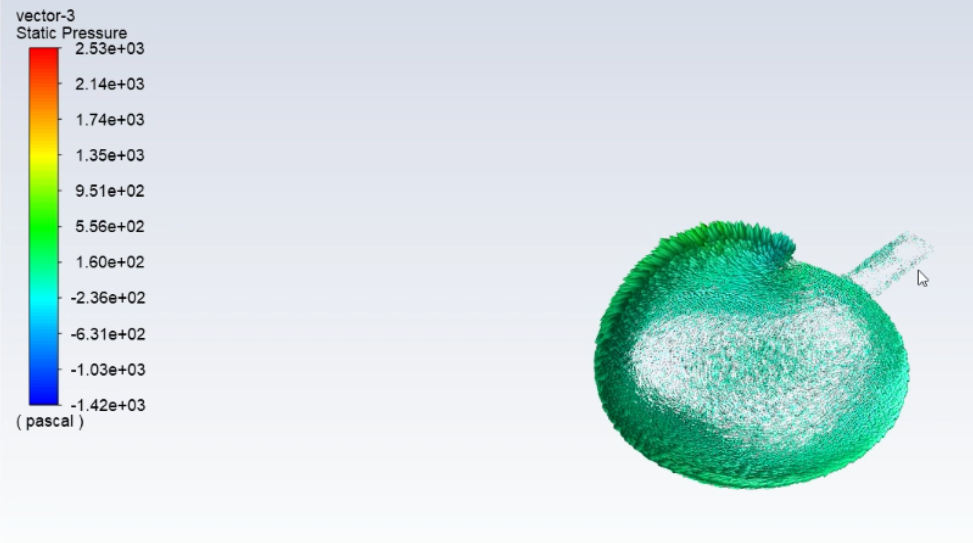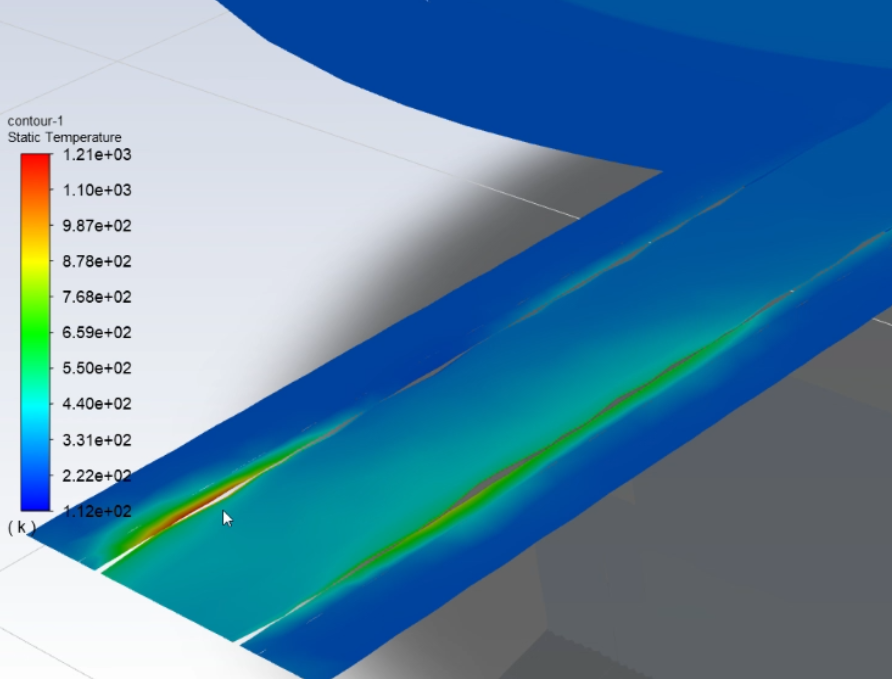## Fluids

•bxddstl
Subscriber

Professor, ,I am a novice. I have a question .I want to create animation .

How do you show on a graph the amount of pressure at a particular x and y coordinates?I think this needs to use a three-dimensional graph, but I can't find a graph in ANSYS Fluent that uses three-dimensional coordinates to represent pressure.Could you help me solve this problem?

In the options shown in the figure below, I select Position on X Axis. Therefore, in plot, X represents direction, Y represents pressure, and X axis represents direction, so it should only represent the direction of a line, that is to say, the plot can only represent the pressure of a line. So I want to ask which line is represented?

•YasserSelima
Subscriber
These are all the cells in the plane. Ignoring the y position and plotting the pressure Vs. X position. n
•bxddstl
Subscriber
Professor, you're saying that x and y are positions, and the density of the dots is pressure, right?nThank you for your help.?.n
•YasserSelima
Subscriber
No, this not what I meant. Sorry if you understand it like this.nIn this plot, X axis is the x.position of each point and Y is the pressure of the point. y.position is not present in this figure.nWhat I tried to say in my earlier comment is that what you see in the figure is not a line. These are discrete points. Every cell in the plane-7 is represented by one point in this figure. n
•bxddstl
Subscriber
Professor, you're saying that every point on an y axis of plot means every point on the y axis of one x position in the object,but y position will not display,right?nThank you for your helpn
•YasserSelima
Subscriber
If I understand you correctly, yes. All the points at the same x location on the plane, appear on one point in the chart because they have the same pressure. n
•bxddstl
Subscriber
Professor，I have another question. In the pressure plot, the vector is dense in some places and sparse in some places, so what does the density of the vector mean?Because the color represents the pressure,the density can not represent the pressure.nAnother question is that in the temperature plot, a small part of the temperature is red color. According to the color -- temperature bar, red represents 1.21e+03, that is, 1210 K. This is obviously impossible.I want to ask why?nThank you for your help.n
•YasserSelima
Subscriber
Fluent creates one vector for every cell. So, the density means there are finer mesh in this area. If you want to see the pressure, select contours of pressure .. now the colour represent the pressure.nnFor the temperature, this means something is wrong ... may be not converged solution.n
•YasserSelima
Subscriber
What is the maximum temperature in your system?n
•bxddstl
Subscriber
Professer,I don't not the maximum temperature. But the inlet flow temperature is 313k and is the maximum temperature the I imoprt to the system. nThank you for your help. n
•YasserSelima
Subscriber
This means your solution did not converge. Can you plot the residuals? n